# Hankel functions

(diff) ← Older revision | Latest revision (diff) | Newer revision → (diff)

Cylinder functions of the third kind. They may be defined in terms of Bessel functions as follows: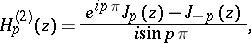whereis not an integer. This implies the important relations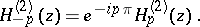Hankel functions are complex for real values of; however,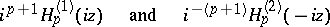are real ifis real and positive. Hankel functions have simple asymptotic representations for large: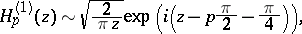The Hankel function of a "half-integral" argument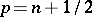can be expressed in terms of elementary functions, in particular: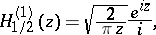These functions were introduced by H. Hankel in 1869.

How to Cite This Entry:
Hankel functions. Encyclopedia of Mathematics. URL: http://encyclopediaofmath.org/index.php?title=Hankel_functions&oldid=17400
This article was adapted from an original article by P.I. Lizorkin (originator), which appeared in Encyclopedia of Mathematics - ISBN 1402006098. See original article